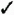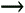# Section 49. About Calc RangesBEFORE YOU BEGIN

45 Copy and Move FormulasSEE ALSO

50 Create a Range

A selected group of cells comprises a range . A range is always rectangular, and it might be a single cell , a row, a column, or several adjacent rows and columns . The cells within a range are always contiguous, but you can select multiple ranges at the same time. You can perform various operations on ranges, such as moving and copying. If, for example, you want to format a row of totals in some way, you first select the range that includes the totals and then apply the format to that range.KEY TERM

Range One or more cells, selected adjacent to each other in a rectangular manner, that you can name and treat as a single entity or group of cells in formulas.

The next figure shows three selected ranges on a sheet. You can describe a range by the cell reference of the upper-left cell of the range (the anchor point ) and the cell reference of the lower-right cell of the range. As you can see from the figure, multiple-celled ranges are designated by listing the anchor point, followed by a colon (:), followed by the range's lower-right cell reference. Therefore, the range that begins at C8 and ends at E12 has the range of C8:E12 . To select more than one range, in case you want to apply formatting or calculations to different areas of your worksheet at once, hold Ctrl while selecting the ranges.KEY TERM

Anchor point One corner of a range of cells; typically, the upper-left cell in a range is considered the anchor point, although any of the four corner cells can be considered an anchor point also.

A sheet can have multiple ranges selected at one time.Keep in mind that a single cell, if selected, can be considered a range. So, D5:D5 is a range composed solely of the cell D5 . In this case, the anchor point is the entire range, which is only one cell.

The true power of Calc shows when you use ranges of cells, as opposed to specifying every individual cell, in formulas. ( 50 Create a Range explains how to name ranges.) Instead of referring to the range F2:G14 , you can name that range MonthlySales and then refer to MonthlySales in your formulas by name.

All the following are valid formulas. Cell references or range names appear throughout the formulas:

`  =(SalesTotals)/NumOfSales   =C4 * 2 - (Rate * .08)   =7 + LE51 - (Gross - Net)  `

When you enter formulas that contain range references, you can either type the full reference or point to the cell reference. If you want to include a complete named range in a formula (formulas can work on complete ranges), select the entire range, and Calc inserts the range name in your formula. Often, finding and pointing to a value is easier than locating the reference and entering it exactly.

If, for example, you are entering a formula, when you get to the place in the formula that requires a cell reference, don't type the cell reference (such as G23 ); instead, point to and click on the cell you want to use in the formula, and Calc adds that cell reference to your formula. If you enter a formula such as =7 + , instead of typing a cell reference of LE51 , you can point to that cell and press Enter to end the formula or type another operator to continue the formula. Immediately after typing the cell reference for you, Calc returns your cell pointer to the formula (or to the Formula bar if you are entering the formula there) so that you can complete the formula.TIP

Range names are absolute. If a formula in one cell refers to a range named Commission , Calc considers the reference to be absolute (see 45 Copy and Move Formulas ). Also, you don't use the dollar sign as you would when making cell addresses absolute (such as \$B\$10 ).

After you assign a name to a range, you don't have to remember that range's address, such as R31 , when you use it in formulas. Suppose that you are creating a large worksheet that spans many screens. If you assign names to cells when you create themespecially to cells that you know you will refer to later during the worksheet's developmententering formulas that use those names is easier. Instead of locating a cell to find its address, you need only type its name when entering a formula that uses that cell.Sams Teach Yourself OpenOffice.org 2, Firefox and Thunderbird for Windows All in One
ISBN: 0672328089
EAN: 2147483647
Year: 2005
Pages: 232
Authors: Greg Perry